15
Oct 15

## Random samples in JS using R functions

For a JavaScript-based project I’m working on, I need to be able to sample from a variety of probability distributions. There are ways to call R from JavaScript, but they depend on the server running R. I can’t depend on that. I need a pure JS solution.

I found a handful of JS libraries that support sampling from distributions, but nothing that lets me use the R syntax I know and (mostly) love. Even more importantly, I would have to trust the quality of the sampling functions, or carefully read through each one and tweak as needed. So I decided to create my own JS library that:

• Conforms to R function names and parameters – e.g. rnorm(50, 0, 1)
• Uses the best entropy available to simulate randomness
• Includes some non-standard distributions that I’ve been using (more on this below)

I’ve made this library public at Github and npm.

Not a JS developer? Just want to play with the library? I’ve setup a test page here.

Please keep in mind that this library is still in its infancy. I’d highly recommend you do your own testing on the output of any distribution you use. And of course let me know if you notice any issues.

In terms of additional distributions, these are marked “experimental” in the source code. They include the unreliable friend and its discrete cousin the FML, a frighteningly thick-tailed distribution I’ve been using to model processes that may never terminate.

11
Dec 14

## Can pregnant women intuit the sex of their children?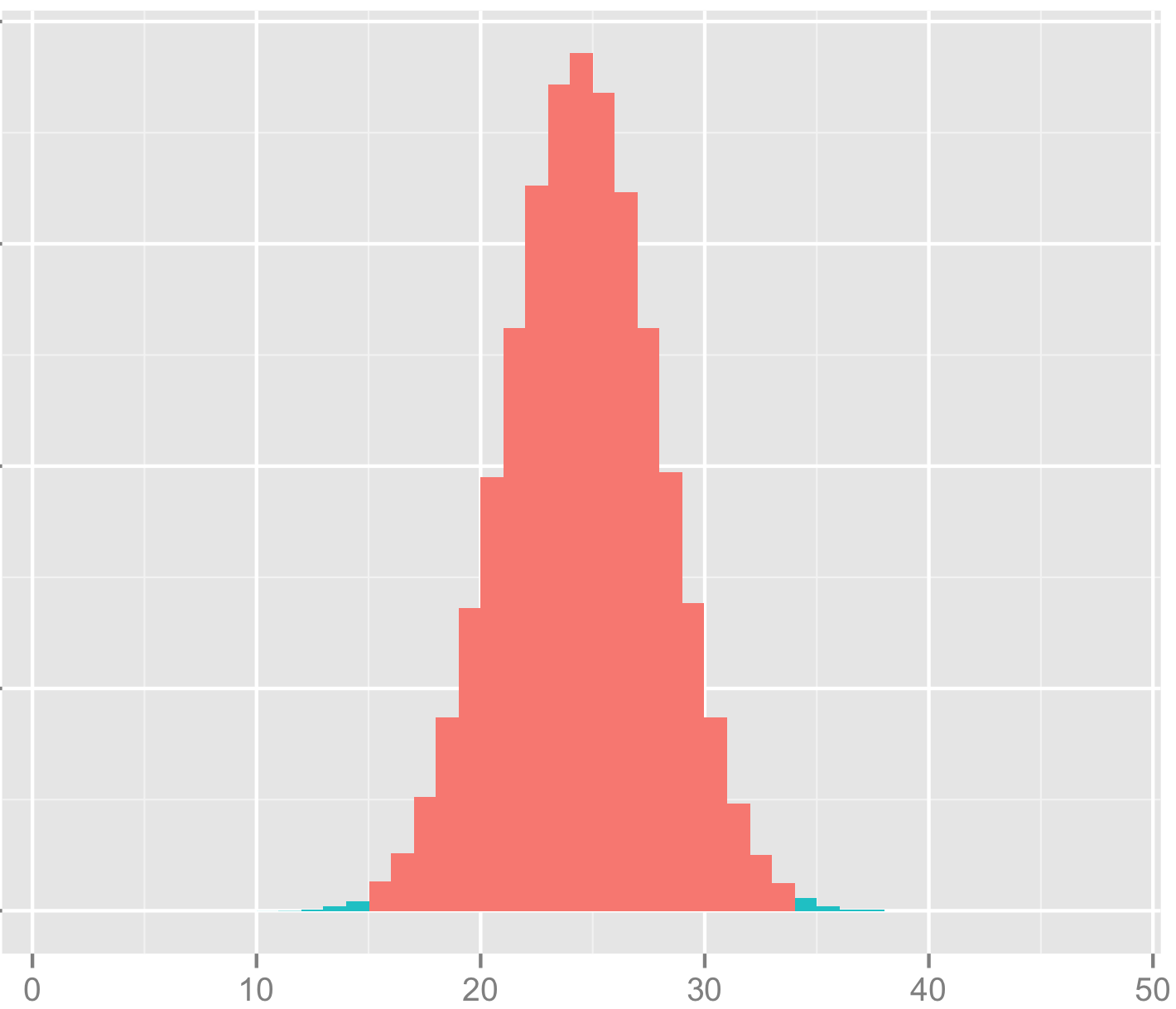“So let’s start with the fact that the study had only 100 people, which isn’t nearly enough to be able to make any determinations like this. That’s very small power. Secondly, it was already split into two groups, and the two groups by the way have absolutely zero scientific basis. There is no theory that says that if I want a girl or if I want a boy I’m going to be better able at determining whether my baby is in fact a girl or a boy.”

– Maria Konnikova, speaking on Mike Pesca’s podcast, The Gist.

Shown at top, above the quote by Konnikova, is a simulation of the study in question, under the assumption that the results were completely random (the null hypothesis). As usual, you’ll find my code in R at the bottom. The actual group of interest had just 48 women. Of those, 34 correctly guessed the sex of their gestating babies. The probability that you’d get such an extreme result by chance alone is represented by the light green tails. To be conservative, I’m making this a two-tailed test, and considering the areas of interest to be either that the women were very right, or very wrong.

The “power” Konnikova is referring to is the “power of the test.” Detecting small effects requires a large sample, detecting larger effects can be done with a much smaller sample. In general, the larger your sample size, the more power you have. If you want to understand the relationship between power and effect size, I’d recommend this lovely video on the power of the test.

As it turns out, Konnikova’s claims notwithstanding, study authors Victor Shamas and Amanda Dawson had plenty of power to detect what turns out to be a very large effect. Adding together the two green areas in the tails, their study has a p-value of about 0.005. This a full order of magnitude beyond the generally used threshold for statistical significance. Their study found strong evidence that women can guess the sex of their babies-to-be.

Is this finding really as strong as it seems? Perhaps the authors made some mistake in how they setup the experiment, or in how they analyzed the results.

Since apparently Konnikova failed not only to do statistical analysis, but also basic journalism, I decided to clean up on that front as well. I emailed Dr. Victor Shamas to ask how the study was performed. Taking his description at face value, it appears that the particular split of women into categories was based into the study design; this wasn’t a case of “p-value hacking”, as Konnikova claimed later on in the podcast.

Konnikova misses the entire point of this spit, which she says has “absolutely zero scientific basis.” The lack of an existing scientific framework to assimilate the results of the study is meaningless, since the point of the study was to provide evidence (or not) that that our scientific understanding lags behind what woman seem to intuitively know.

More broadly, the existence of causal relationships does not depend in any way on our ability to understand or describe (model) them, or on whether we happen to have an existing scientific framework to fit them in. I used to see this kind of insistence on having a known mechanism as a dumb argument made by smart people,  but I’m coming to see it in a much darker light. The more I learn about the history of science, the more clear it becomes that the primary impediment to the advancement of science isn’t the existence of rubes, it’s the supposedly smart, putatively scientific people who are unwilling to consider evidence that contradicts their worldview, their authority, or their self-image. We see this pattern over and over, perhaps most tragically in the unwillingness of doctors to wash their hands until germ theory was developed, despite evidence that hand washing led to a massive reduction in patient mortality when assisting with births or performing operations.

Despite the strength of Shamas and Dawson’s findings, I wouldn’t view their study as conclusive evidence of the ability to “intuit” the sex of your baby. Perhaps their findings were a fluke, perhaps some hidden factor corrupted the results (did the women get secret ultrasounds on the sly?). Like any reasonable scientist, Shamas wants to do another study to replicate the findings, and told me that has a specific follow-up in mind.

Code in R:

trials = 100000
results = rep(0,trials)
for(i in 1:trials) {
results[i] = sum(sample(c(0,1),48,replace=T))
}

extremes = length(results[results<=14]) + length(results[results>=34])
extremes/trials

dat <- data.frame( x=results, above=((results <= 14) | (results >= 34)))
library(ggplot2)
qplot(x,data=dat,geom="histogram",fill=above,breaks=seq(1,48))


1
Sep 14

## Labor day distribution fun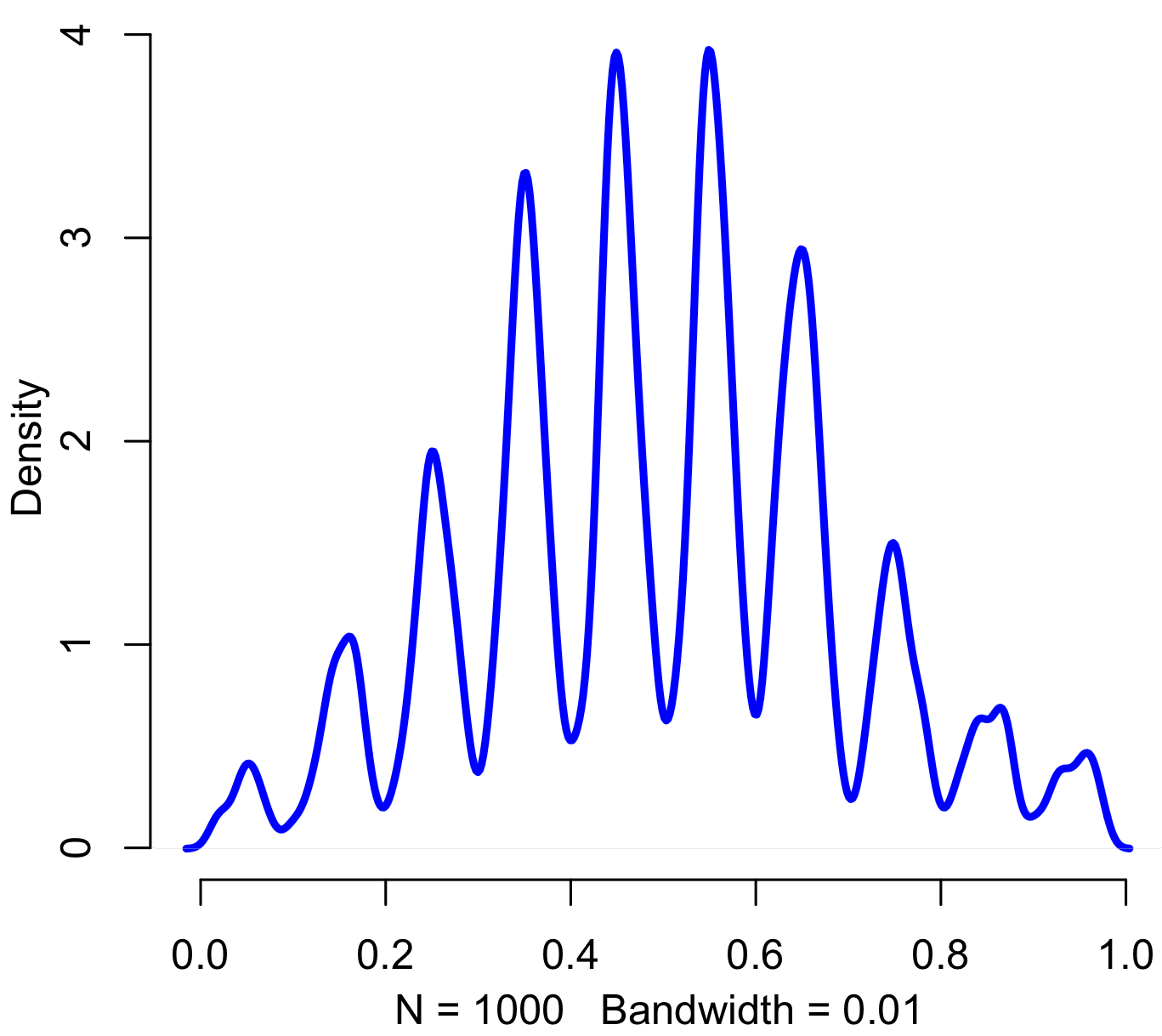Pinned, entropy augmented, digitally normal distribution, of no particular work-related use and thus perfectly suitable for today. Code in R:

iters = 1000
sd = 2
precision = 20

results = rep(0,iters)

for(i in 1:iters) {
x = floor(rnorm(20,5,sd) %% 10)
results[i] = paste(c('.',x),sep="",collapse="")
}

results = as.numeric(results)

plot(density(results,bw=.01),col="blue",lwd=3,bty="n")


9
Sep 13

## The week in stats (Sept. 9th edition)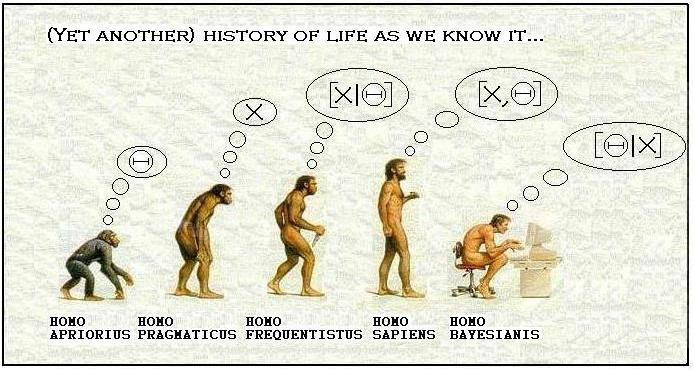3
Jul 13

## The hat trickIn his book Quantum Computing Since Democritus, Scott Aaronson poses the following question:

Suppose that you’re at a party where every guest is given a hat as they walk in. Each hat has either a pineapple or a watermelon on top, picked at random with equal probability. The guests don’t get to see the fruit on their own hats, but they can see all of the other hats. At no point in the evening can they communicate about what’s on their heads. At midnight, each person predicts the fruit on their own hat, simultaneously. If more than 50% of the guests get the correct answer, they’re given new Tesla cars. If less than 50% of the guests get it right, they’re given anxious goats to take care of. What strategy (if any) can they use to maximize their chances of winning the cars?

Answer: there is no strategy that works.

Kidding! Of course there’s a strategy, as you can tell by the length of this post. Did you come up with any ideas? At first glance, it seems like the problem has no solution. If you can’t communicate with the other party goers, how can you find out any information about the fruit on your own head? Since each person was independently given a pineapple or a watermelon with equal probability, what they have on their heads tells you nothing about what you have on your head, right?

My own initial strategy, after considerable (but not enough!) thought, was to bet on regression to the mean. Suppose you see 7 pineapples and 2 watermelons. The process of handing out hats is more likely to generate a pineapple/watermelon ratio of 7 to 3 than 8 to 2 (it’s most likely to generate an equal number of each type, with every step away from a 5/5 ratio less and less likely). Thus, I figured it would be best to vote that my own hat moved the group closer towards the mean. Following my strategy, we all ended up with goats. What did I do wrong?

The key to solving this problem is to realize that the initial process for handing out hats is irrelevant. All that matters is that, from the perspective of a given person, they are a random sampling of 1 from a distribution that is known to have either 7 pineapples and 3 watermelons, or 8 pineapples and 2 watermelons. Thus, each person knows that the probability a randomly sampled guest will have a pineapple on their head is somewhere between 70% and 80%. More precisely, it’s either 70% or 80%. In any case, so long as every person votes for themselves being in the majority, then the majority of guests will be voting that they are in the majority.

I simulated this strategy using parties of different sizes, all of them odd (to avoid the issue of having and equal number of each hat type). Here’s the plot, with each point representing the mean winning percentage with 500 trials for each group size. As always, you can find my code at the end of the post.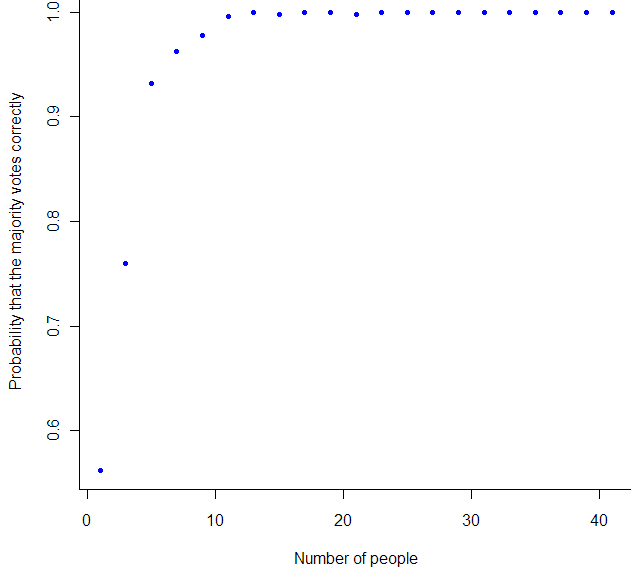As you can tell from the chart, once we have 11 or more guests, it’s highly likely that we all win Teslas.

One way to look at this problem is through the lens of the anthropic principle. That is, we need to take into account how what we observe gives us information about ourselves, irrespective of the original process that made each of our hats what they are. What matters is that from the perspective of each party goer, their view comprises a random sampling from the particular, finite distribution of pineapples and watermelons that was set in stone once everyone had entered the room. In other words, even if the original probably of getting a pineapple was 99%, if you see more watermelons than pineapples, that’s what you should vote for.

This problem, by the way, is related to Condorcet’s Jury Theory (featured on the most recent episode of Erik Seligman’s Math Mutation podcast). Condorcet showed, using the properties of the binomial distribution, that if each juror has a better than 50% chance of voting in accordance with the true nature of the defendant, then the more jurors you add, the more likely the majority vote will be correct. And vice versa. Condorcet assumed independence, which we don’t have because our strategy ensures that every person will vote the same way, so long as the difference between types of hats is more than 2.

# Code by Matt Asher for StatisticsBlog.com
# Feel free to modify and redistribute, but please keep this header
set.seed(101)
iters = 500
numbPeople = seq(1, 41, 2)
wins = rep(0, length(numbPeople))

cntr = 1
for(n in numbPeople) {
for(i in 1:iters) {
goodGuesses = 0
hats = sample(c(-1,1), n, replace = T)
disc = sum(hats)
for(h in 1:n) {

personHas = hats[h]
# Cast a vote based on what this person sees
personSees = disc - personHas

# In case of a tie, the person chooses randomly.
if(personSees == 0) {
personSees = sample(c(-1,1),1)
}

personBelievesHeHas = sign(personSees)

if(personBelievesHeHas == personHas) {
goodGuesses = goodGuesses + 1
break
}

}

if(goodGuesses > .5) {

# We win the cars, wooo-hooo!
wins[cntr] = wins[cntr] + 1
}
}

cntr = cntr + 1
}

winningPercents = wins/iters

plot(numbPeople, winningPercents, col="blue", pch=20, xlab="Number of people", ylab="Probability that the majority votes correctly")


30
May 13

## Uncovering the Unreliable Friend Distribution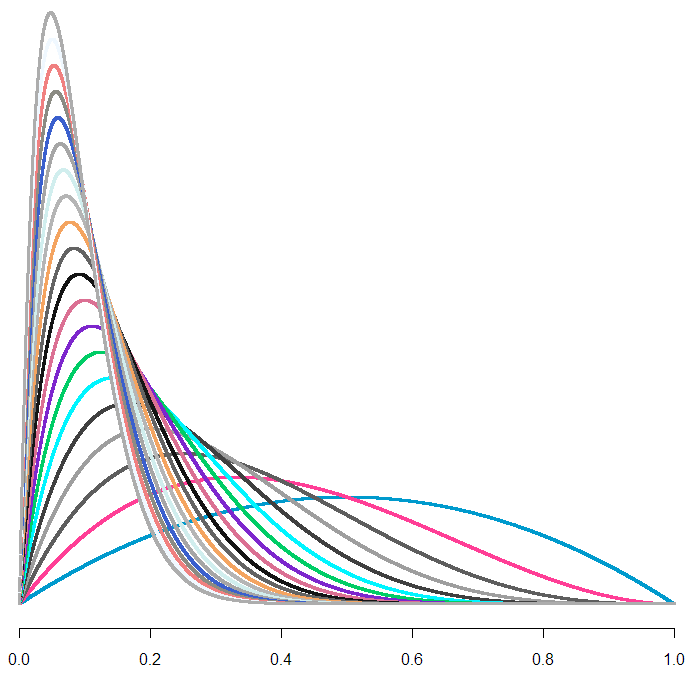Head down to your local hardware store and pick up a smoke detector. Pop off the cover and look inside. You’ll see a label that mentions Americium 241, a radioactive isotope. Put on your HEV suit, grab a pair of tweezers and a fine-tipped pen, and remove the 0.3 millionths of a gram of Americium. If you need reading glasses, now might be a good time to put them on. Pick out one of atoms and label it with an X. Now watch closely. Sooner or later, it will spit out an Alpha particle.

Just how long will you have to wait? Decay rates are measured in half-lives, which is the amount of time needed for half of the particles to decay (any particular atom has a 1/2 chance of decaying in this time as well). The stated half-life for this isotope is 432 years, and your waiting time will follow an exponential distribution. The strange, oddly beguiling quality about this distribution is that the conditional probabilities remains constant. In other words, no matter how long you’ve waited, there’s still a 1 in 2 chance that your Americium isotope will decay in the next 432 years. Waiting for an exponentially distributed event to happen leads to an odd feeling, at least for me. The longer you wait, the more you “expect” the event to happen soon, even knowing that your expected wait time never changes. I wrote about that feeling previously, and created an exponential timer you can try out for yourself. I would suggest setting it to less than 432 years.

Cranking uncertainty up to 11
Recently, as I waited patiently for my own particle of Americium to give up its Alpha, I got to thinking about conditional uncertainty. No matter how long we wait for our event, we never get any smarter about when it will happen. But we don’t get any dumber, either. Would it be possible, I wondered, to build a kind of “super-exponential” distribution, where the longer we wait, the less we know. In other words, can we take our level of uncertainty up to 11?

Imagine the following scenario: first we sample from a standard uniform distribution, which gives us a number somewhere between 0 and 1. Call this number $U$. Then we take $U$ (without looking at it!), and plug it into the exponential distribution as the parameter $\lambda$. This gives us a random variable with a mean waiting time of $\frac{1}{U}$ for the first occurrence. (Note that this mean isn’t the same as the half-life, which is actually the median. To convert from mean to half-life, multiply by the natural log of 2).

My prediction was that this method would increase the overall level of uncertainty about our waiting time, and, even worse, make our uncertainty grow over time. Why? The longer we’ve waited, I figured, the more likely our (presumed) $\lambda$ will be small, which in turn means the expectation and variance of our exponential waiting time grow, widening our confidence intervals.

At this point, I had the vague feeling that this probability distribution should already exist as a known thing, that it may even be a version of something I’ve encountered before. Another way to look at the exponential is in terms of the failure rate, or, conversely, the survival rate. When Ed Norton, the un-named narrator of Fight Club (I know, I know), says that “on a long enough timeline, the survival rate for everyone drops to zero,” this is what he means. Only Norton is referring to the cumulative survival rate, whereas it’s usually most interesting to look at the instantaneous (or marginal, for my economist friends) rate. For the exponential this rate is constant, ie flat. There is a distribution specifically crafted to let you simulate failure rates when the rate itself is variable, it’s called the Weibull. It can be used to model products whose expected durability increases with time (note that we are not saying the product becomes more durable over time, but that the fact that it has survived tells us that it is highly durable). Did I just rediscover the Weibull, or one it’s friends in the same family of extreme distributions?

Before breaking out my great big Compendium of Probability Distributions, I dove right in with a quick Monte Carlo simulation. As with all my posts using R, you’ll find the code at the end of this post.

A wave of plots
Here’s the histogram for our sample, with the rightmost tail chopped off (because your screen, unlike mine, is just too damn small):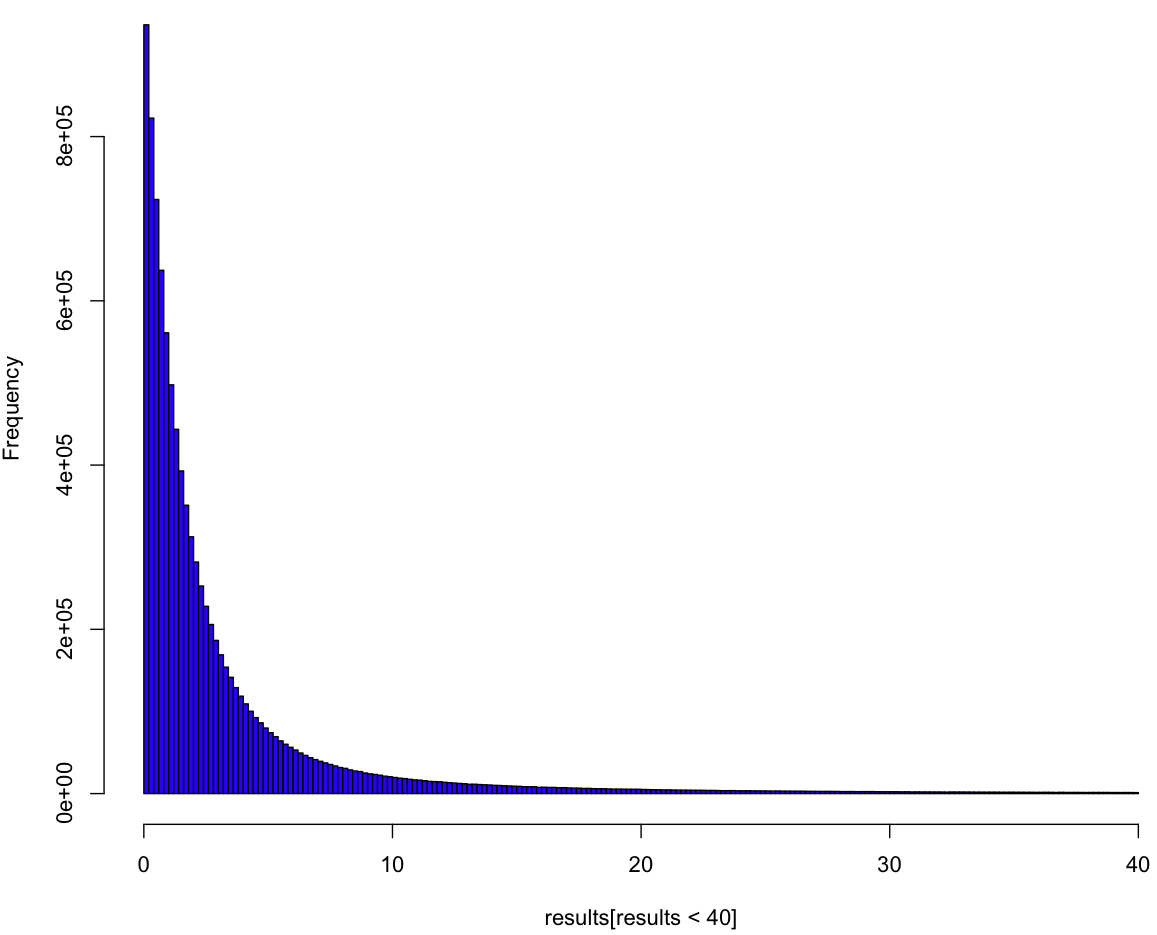So it looks like a variant of the exponential, but this plot doesn’t tell us much. To really understand the distribution we have to see it as if we were inside the distribution, waiting for the event to happen. All we know is the process, and we have to come up with a guess about our distribution curve conditional on how long we’ve waited so far. In order to understand this curve, we first need to make a guess about $\lambda$, which is to say $U$. Can we put a probability distribution on $U$ given how long we’ve been waiting so far? Yes, we most certainly can! And, because our prior distribution on $U$ is uniform (of course), our posterior is our likelihood. Here’s what our (posterior) curves look like: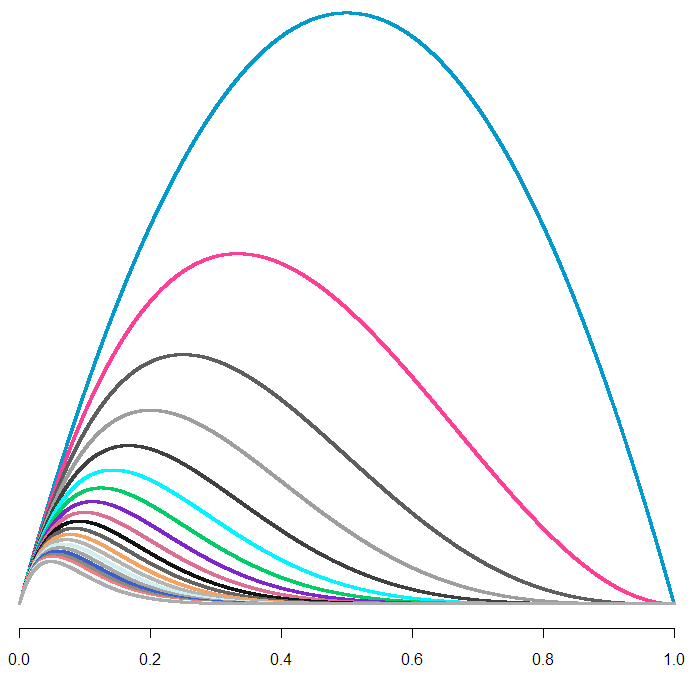Each curve is a probability distribution on our belief about $U$. In other words, the peaks represent what we believe to be the most likely value for $U$, given how long we’ve waited so far. The biggest curve is our distribution for $U$ after waiting for one unit of time (let’s just call them “minutes”). As you can tell, if we continue to wait, our maximum likelihood estimate (MLE) for $U$ shifts left, and it looks like our curve flattens out. But wait! Each of these curves has a different area. To treat them like a true probability distribution, we should normalize each of the areas to one. Here’s what those same curves look like after normalization:From this handsome chart (the same one from the beginning of the post), we can tell that expected range of values for $U$ is narrowing, not broadening. So could our uncertainty be decreasing along with our wait, as we hone in on the true value of $U$? Let’s take a look at what happens to our additional wait time as time passes.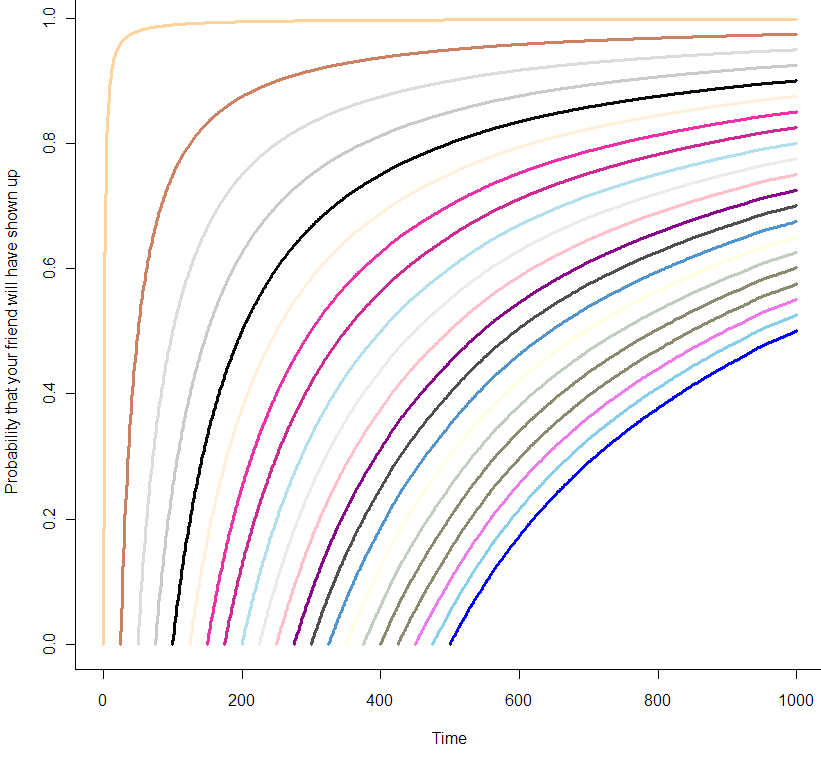You can think of these curves as the chance that your friend will show up in the coming minutes, given how long you’ve already been waiting. At the very beginning of your wait, modeled by the orange curve at the far left, you can be almost certain that your friend will show up in the next 10 minutes. But by the time you’ve been waiting for 500 minutes, as seen in the blue curve at the far right, you are only 50% sure that she will show up in the next 500 minutes. Are those probabilities exact? It seems like it, but let’s zoom in on the first 25 minutes: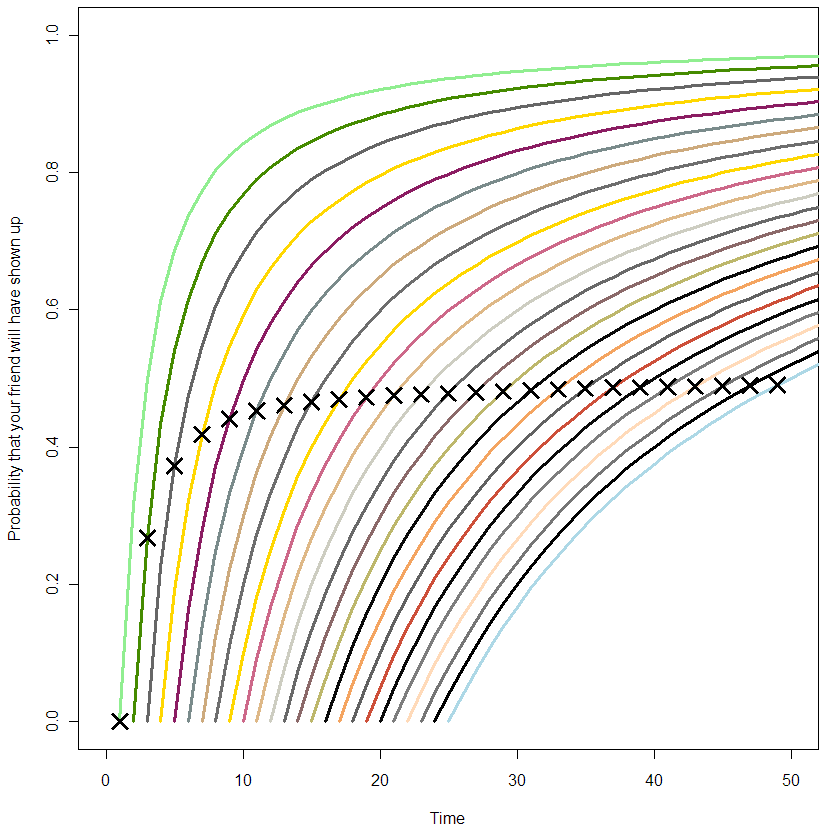The X’s represent the median time for your friend’s arrival. If this was always equal to your wait time so far, all of the X’s would be in a straight line at 0.5. From this plot, it’s clear that this is not the case from the beginning, but only becomes so as you wait longer. So what have we got here? At this point I’m at the limit of what I can get out of Monte Carlo. It’s time to do math! (or not, feel free to skip this next section).

The formula
To get the pdf for this distribtion, I start by noting that if we had two possible choices for $\lambda$ with a one-half chance each of being picked, then the probability our waiting time would be less than $x$ would be:

$P(t < x) = \frac{1}{2}(1 - e^{-x \lambda_1}) + \frac{1}{2} (1 - e^{-x \lambda_2})$

where $(1 – e^{-x \lambda_i})$ is the cumulative distribution function (CDF) of the exponential distribution with parameter $\lambda_i$. If you really know your exponential, you may have noticed some similarities with the hyperexponential distribution, but we’re gonna take it to the limit, and create a kind of hyper-hyperexponential. More generally, for a sample of $\lambda_i$:

$P(t < x) = \frac{1}{n} \sum_{i=1}^n (1 - e^{-\lambda_i x})$ Since the $\lambda_i$ are uniformly distributed, the more of them we sample, the more our order statistics are going to look like $(\frac{1}{n}, \frac{2}{n}, \frac{3}{n}... \frac{n}{n})$ where our sample size is n (proof is left as an exercise for you, my dear reader). $P(t < x) = \frac{1}{n}\sum_{i=1}^n (1 - e^{-xi/n})$

Ready to take it to the limit?

$\lim_{n \rightarrow \infty} \frac{1}{n}\sum_{i=1}^n (1 – e^{-xi/n}) = \int_0^1 1 – e^{xt} dt$

Solving this integral, we get:

$F(t) = \frac{e^{-t} + t – 1}{t}$

Did we get it right?
Maybe you trust my math, maybe you don’t and skimmed over the last section. Either way, let’s see how well the math matches the data. Here I’ve plotted the log of the observed (Monte Carlo) density versus what the math says it should be: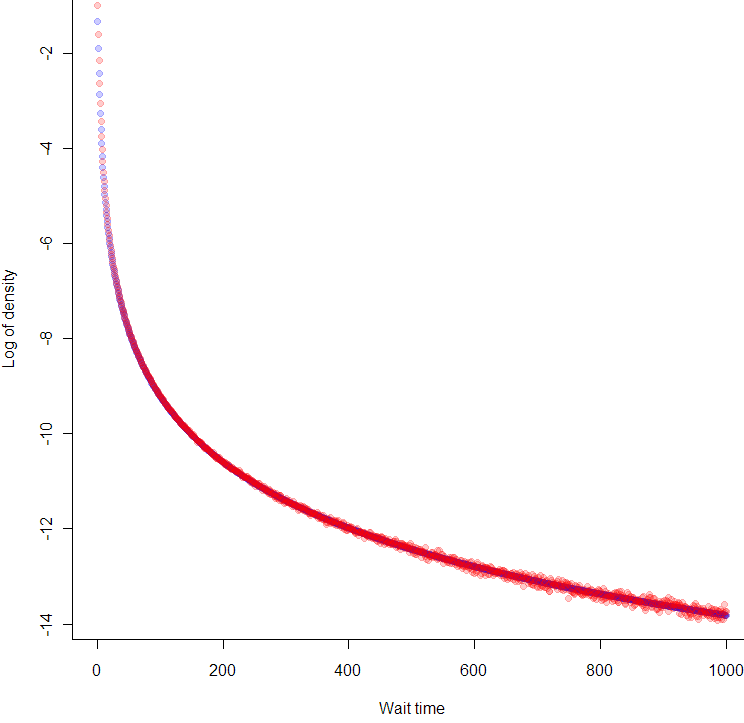Looks like we nailed it, no? But wait, why are the blue points at the beginning of the curve in between the red points? That’s because we took the differences between points on the empirical CDF, so each density reading is really in-between the true pdf values. So far as Monte Carlo confirmation goes, it doesn’t get much better than this.

Introducing, the Unreliable Friend Distribution!
So far as I can tell, other than the hyperexponential, which is merely similar and more limited, this is a brand new distribution. Have you ever been waiting for someone, and the more they make you wait, the more you suspect they’ve forgotten about you completely? In that person’s honor, I’m calling this the Unreliable Friend Distribution (UFD).

As seems appropriate for such a distribution, the expected wait time for the UFD is infinite. Which means that no matter how late your unreliable friend shows up, you should be grateful that he came early.

The code:

# Code by Matt Asher for StatisticsBlog.com
# Feel free to modify and redistribute, but please keep this header

set.seed(943) #I remembered this time!

# Initial MC sampling
trials = 10^7
results = rexp(trials, runif(trials))

# Plot of liklihood curves for U based on waiting time
# colr = sample(colours(), 1000, replace=T)

lik = function(p, t){
return((1 - p)^(t-1)*p)
}

# x-values to plot
p = seq(0,1,0.0001)

# Waiting times
t = 1:20

dataMatrix = matrix(nrow=length(t), ncol=length(p))

for(i in t) {
dataMatrix[i,]=lik(p,rep(i+1,length(p)))
}

plot(p, dataMatrix[1,], col=colr, pch=".", cex=3, bty="n" )

for(i in 2:max(t)) {
points(p, dataMatrix[i,], col=colr[i], pch=".", cex=3)
}

# Let's standardize the area of each curve
standardMatrix = dataMatrix/rowSums(dataMatrix)

plot(p, standardMatrix[1,], col=colr, pch=".", cex=3, bty="n", ylim=c(0,max(standardMatrix)))

for(i in 2:max(t)) {
points(p, standardMatrix[i,], col=colr[i], pch=".", cex=3)
}

# Find wait time curves conditional on having waited t minutes

# We need tail probabilities, let's find them!
t = 0:1000
tailP = rep(0,max(t))
for(i in t) {
tailP[(1+i)] = length(results[results>i])/trials
}

show = seq(1,25,1)

# Blank Plot
plot(0,0,col="white", xlim = c(0,2*max(show)), ylim = c(0, 1), ylab="Probability that your friend will have shown up", xlab="Time")

for(i in show) {
# Normalizing the probabilies so that tailP[i] = 1
tmp = tailP[(i+1):(max(t)+1)]
tmp = tmp * 1/tmp
tmp = 1-tmp

print(length(tmp[tmp<.5]))

# par(new = TRUE)
lines(i:(max(t)), tmp, col=sample(colours(), 1), lwd=3)

# Find the index of the closest tmp to tmp[i]
xloc = which.min(abs(tmp[i] - tmp))

# Put a point where we cross time 2t on the curve
points(i+xloc-1, tmp[i], pch=4, col="black", cex=2, lwd=3)

}

plot(0,0,col="white", xlim = c(0,100), ylim = c(0, 0.25))

t = 1:20

tmp = results[resultsi]), xlim = c(0,100), ylim = c(0, 0.25), col=colr[i], cex=3)
}

tpdf = function(x) {
toReturn = (-x*exp(-x)+1-exp(-x))/x^2
return(toReturn)
}

tF = function(x) {
toReturn = (exp(-x) + x - 1)/x
return(toReturn)
}

lengths = rep(0,1000)
for(i in 0:1000) {
lengths[(i+1)] = length(results[results>i])
}

empericalF = 1 - (lengths/trials)
empericalf = diff(empericalF)

# Because this the the perfect size for the dots!
plot(log(tpdf(1:1000)), col=rgb(0,0,1,.2), pch=20, cex=1.3728, xlab="Wait time", ylab="Log of density")
points(log(empericalf), col=rgb(1,0,0,.2), pch=20, cex=1.3728)


11
Apr 13

## High Obesity levels found among fat-tailed distributions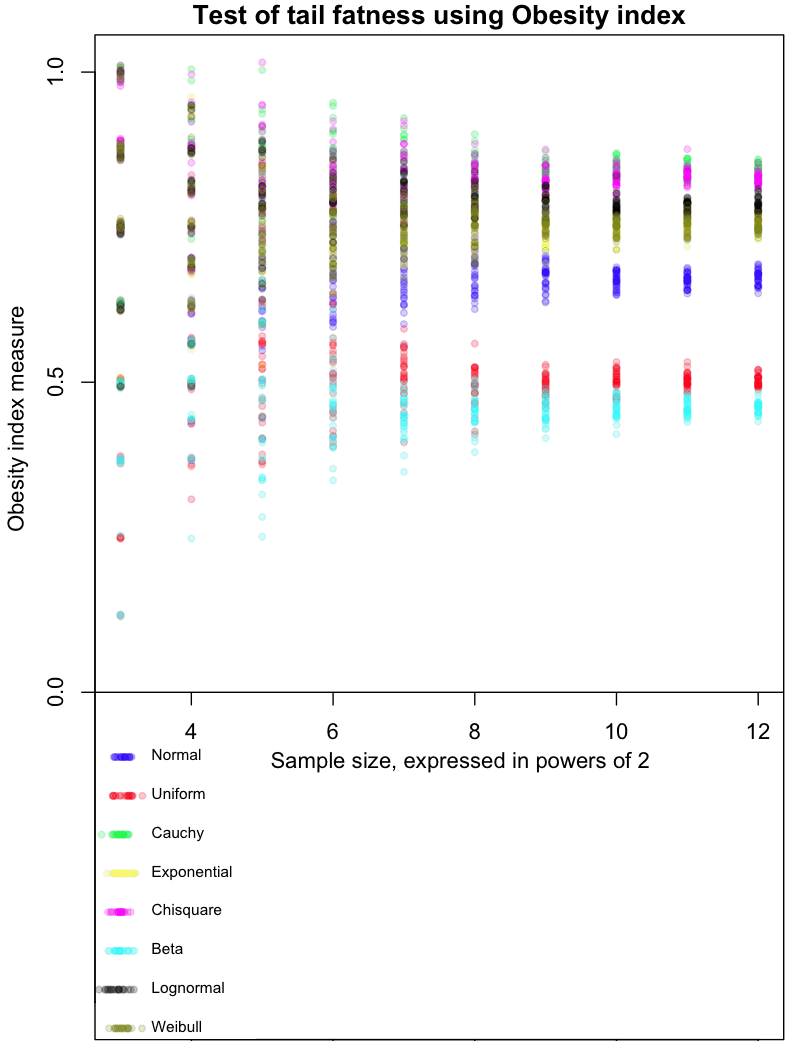In my never ending quest to find the perfect measure of tail fatness, I ran across this recent paper by Cooke, Nieboer, and Misiewicz. They created a measure called the “Obesity index.” Here’s how it works:

• Step 1: Sample four times from a distribution. The sample points should be independent and identically distributed (did your mind just say “IID”?)
• Step 2: Sort the points from lowest to highest (that’s right, order statistics)
• Step 3: Test whether the sum of the smallest and greatest number is larger than the sum of the two middle.

The Obesity index is the probability that the sum of these end points is larger than the sum of the middle numbers. In mathy symbols:

$Ob(X) = P (X_1 + X_4 > X_2 + X_3 | X_1 \leq X_2 \leq X_3 \leq X_4), X_i~IID$

The graph at the top of this post shows how the Obesity index converges for different distributions. As always, I’ve included my R code at the end of this article, so you can run this simulation for yourself (though, as usual, I forgot to set a random seed so that you can run it exactly like I did).

The dots in the graph represent the mean results from 8, 16, 32, and so on, up to 4096 trials from each of the distributions I tested. Note that each trial involves taking 4 sample points. Confused? Think of it this way: each sample of 4 points gives us one Bernoulli trial from a single distribution, which returns a 0 or 1. Find the average result after doing 4096 of these trials, and you get one of the colored dots at the far right of the graph. For example, the red dots are averages from a Uniform distribution. The more trials you do, the closer results from the Uniform will cluster around 0.5, which is the “true” Obesity value for this distribution. The Uniform distribution is, not coincidentally, symmetric. For symmetric distributions like the Normal, we only consider positive values.

The graph gives a feel for how many trials would be needed to distinguish between different distributions based on their Obesity index. I’ve done it this way as part of my Grand Master Plan to map every possible distribution based on how it performs in a variety of tail indices. Apparently the Obesity index can be used to estimate quantiles; I haven’t done this yet.

My initial impressions of this measure (and these are very initial!) are mixed. With a large enough number of trials, it does a good job of ordering distributions in a way that seems intuitively correct. On the other hand, I’d like to see a greater distance between the Uniform and Beta(0.01, 0.01) distribution, as the latter is an extreme case of small tails.

Note that Obesity is invariant to scaling:

$Ob(x) = Ob(k*X)$

but not to translations:

$Ob(X) \neq Ob(X+c)$

This could be a bug or a feature, depending on what you want to use the index for.

Extra special karma points to the first person who comes up with a distribution whose Obesity index is between the Uniform and Normal, and that isn’t a variant of one I already tested.

Here’s the code:

# Code by Matt Asher for StatisticsBlog.com
# Feel free to redistribute, but please keep this notice

# Create random varaibles from the function named in the string
generateFromList = function(n, dist, ...) {
match.fun(paste('r', dist, sep=''))(n, ...)
}

# Powers of 2 for testAt
testAt = 3:12
testAtSeq = 2^testAt
testsPerLevel = 30

distros = c()

distros = 'generateFromList(4,"norm")'
distros = 'generateFromList(4,"unif")'
distros = 'generateFromList(4,"cauchy")'
distros = 'generateFromList(4,"exp")'
distros = 'generateFromList(4,"chisq",1)'
distros = 'generateFromList(4,"beta",.01,.01)'
distros = 'generateFromList(4,"lnorm")'
distros = 'generateFromList(4,"weibull",1,1)'

# Gotta be a better way to do this.
dWords = c("Normal", "Uniform", "Cauchy", "Exponential", "Chisquare", "Beta", "Lognormal", "Weibull")

par(mar=c(4,5,1.5,.5))
plot(0,0,col="white",xlim=c(min(testAt),max(testAt)), ylim=c(-.5,1), xlab="Sample size, expressed in powers of 2", ylab="Obesity index measure", main="Test of tail fatness using Obesity index")

abline(h=0)

colorList = list()
colorList[]=rgb(0,0,1,.2)
colorList[]=rgb(1,0,0,.2)
colorList[]=rgb(0,1,0,.2)
colorList[]=rgb(1,1,0,.2)
colorList[]=rgb(1,0,1,.2)
colorList[]=rgb(0,1,1,.2)
colorList[]=rgb(0,0,0,.2)
colorList[]=rgb(.5,.5,0,.2)

# Create the legend
for(d in 1:length(distros)) {
x = abs(rnorm(20,min(testAt),.1))
y = rep(-d/16,20)
points(x, y, col=colorList[[d]], pch=20)
text(min(testAt)+.25, y, dWords[d], cex=.7, pos=4)

}

dCounter = 1
for(d in 1:length(distros)) {
for(l in testAtSeq) {
for(i in 1:testsPerLevel) {
count = 0
for(m in 1:l) {

# Get the estimate at that level, plot it testsPerLevel times
x = sort(abs(eval(parse( text=distros[dCounter] ))))
if ( (x+x)>(x+x) ) {
count = count + 1
}
}

# Tiny bit of scatter added
ratio = count/l
points(log(l, base=2), ( ratio+rnorm(1,0,ratio/100)), col=colorList[[dCounter]], pch=20)
}
}

dCounter = dCounter + 1
}



18
Mar 13

## Review of Mathematica 9 and R-link

VIDEO TRANSCRIPT: Hello, this is Matt Asher from StatisticsBlog.com. I’m going to be reviewing Mathematica 9, from Wolfram Research. In particular, I’ll be focusing on using it with R and to do Monte Carlo simulations and other statistical work. You can find a full transcript of this video at my blog, including the source code and links to all of the webpages I mention.

Before I begin I’d like to thank Jeff Hara and Andy Ross from Wolfram for their time. Also thanks to the folks at the Mathematica Stack Exchange, who helped with a couple of my questions.

I’m going to get started with a blank notebook. I’m going to clear out all of the variables that exist. I’ve found sometimes that if you have existing variables that can cause problems.

ClearAll["Global*"]


After each line I’m hitting Shift+Enter to run the command, if you just hit enter Mathematica won’t run things yet.

So I’ve cleared my variables and I’m going to run

Needs["RLink"]


which will bring in the link between Mathematica and R.

InstallR[]


I’m going to make sure it’s installed.

REvaluate["R.Version()"]


And then I’m going to run a test command here to make sure everything is up and running. As you can see this is the version of R I’m running and the connection succeeded.

Note that the free version of Mathematica, the evaluation version, doesn’t come with support for R, so if you want to test out Mathematica’s and its interactions with R you either have to have to buy the full version or maybe if you call up or contact Wolfram they’d be willing to let you have a free evaluation version that is full and allows you to test out R.

So how does the interface between R and Mathematica work?

Basically, you can run operations in R, then store the results to variables in R. You can also pass data types back and forth between R and Mathematica.

Here I’m setting a variable and this variable is set in R, not in Mathematica

RSet["hal", 9000]


So if I were to type just

hal


There is no response back. This is Mathematica’s way of saying that the variable is undefined or that it doesn’t know what to do with your input. So to get back information from R we have to use:

REvaluate["hal"]


We are putting “hal” in quotes so we are parsing this in R and not in Mathematica.

For example we can do things like grab a dataset from R

iris = REvaluate["iris"]


I’m grabbing the famous “iris” dataset in R and I am pulling it into Mathematica.

or I could do things like evaluate a command in R:

REvaluate["det(matrix(sample(-50:49),nrow=10))"]


and bring back the results. This grabs the determinant of a random matrix.

We can even do things like create our own functions in R, and this gets put into a variable in Mathematica.

perfectSample = RFunction["function(n, dist, ...) match.fun(paste('q', dist, sep=''))((1:n) / (n+1), ...)"]


This function creates a perfect sample of the length that you specify of a particular distribution. Then we can call that function directly in Mathematica.

perfectSample[100, "pois", 10]


and the results are returned.

Of course, if we just wanted to do things in R, we would be continuing to just use R, instead of having to learn this new interface between R and Mathematica. So then what can we do in Mathematica that goes beyond what we can easily do in R?

One of the biggest advantages to using Mathematica is that you get access to tools for creating interactive plots and simulations that can be changed on the fly.

I’m going to do an example using the Benini Distribution, which, according to Wolfram’s web page, can be used to model the weight of cats.

So to do that, what I’m going to do is use the Mathematica command “Manipulate”

Manipulate[Block[{data, dist, kmd},
data = RandomVariate[dist = BeniniDistribution[\[Alpha],\[Beta], \[Sigma]], n];
kmd = KernelMixtureDistribution[data, h, MaxMixtureKernels -> All];
Plot[{PDF[dist, x], PDF[kmd, x]}, {x, 0, xRng},
PlotRange -> Automatic]], {{\[Alpha], 1}, .01, 5}, {{\[Beta], 1}, 0.01,
5}, {{\[Sigma], 1}, .01, 2}, {{n, 100}, {10, 100, 1000, 10000}}, {{xRng, 5}, 1,
10}, {{h, .5}, 0.01, 1}]


And then I get back the results and what I’ve got here is a live Monte Carlo simulation where I am specifying the different parameters of the distribution and I’m also specifying how many variates that I’m creating. This is the smoothing, the kernel bandwidth that I am adjusting.

And I can adjust the size of it here. Make it bigger. And do all of these adjustments on the fly.

As you can see, you’ve got some good power here for generating interactive plots and simulations. You can do these natively in Mathematica, or you do live manipulation of results coming from R. This example comes from the Mathematica guys:

mathematicaRPlotWrapper = RFunction["function(filename, plotfun){
pdf(filename)
plotfun()
dev.off()
}"];

Clear[getRPlot];
getRPlot[plotFun_RFunction] :=
With[{tempfile = FileNameJoin[{$TemporaryDirectory, "temp.pdf"}]}, If[FileExistsQ[tempfile], DeleteFile[tempfile]]; mathematicaRPlotWrapper[tempfile, plotFun]; If[! FileExistsQ[tempfile], Return[$Failed]];
Import[tempfile]];

myFun[t_] :=
Show[#, ImageSize -> Medium, PlotRange -> All] &@
getRPlot[RFunction["function(){
x<- seq(1," <> ToString@t <> ",0.1)
y<- sin(x)
plot(y~x)
}"]]


What's going to happen here is I am calling an R function, doing all of my calculations, bringing them back into Mathematica.

I forgot the "Manipulate" part:

Manipulate[myFun[t], {t, 2, 10}]


So here we go. And what's happening is everything is being sent to R for processing then coming all the way back to Mathematica. As you can see even though we are making that round trip the results are coming back at a good pace, it's almost instantaneous this particular example.

What about speed though, more generally?

I tried creating some random variates like I did in my examination of JavaScript versus R. So I'm going to create 10 million random variates from a Normal distribution

Timing[data = RandomVariate[NormalDistribution[], 10^7];]


and that takes about a quarter of a second, a little bit more, which is about twice as fast as R for generating those.

But then let's look at more of a worst-case scenario, a bunch of nested loops.

Timing[
l = 0;
For [i = 0, i < 10^2, i = i + 1,
For[j = 0, j < 10^2, j = j + 1,
For[k = 0, k < 10^2, k = k + 1,
l = l + 1
]
]
]
]


Three of them, a total of a million loops it's going through, and this takes about 1.2 seconds, and it will increase by an order of magnitude if I add an order of magnitude in here. That is slow, it's about twice as slow as the same code would take to run in R, a language not known for it's speed with loops. Of course, this is generally speaking not what you want to do if you want to run fast code. Mathematica itself on their website advises against using these kinds of procedural codes.

But at the same time I've found that there are times when there really is no other way to do things, especially if you are doing simulations with a bunch of objects that take place over time, that are iterative, in which case you do need a programming structure that is like this, so you'll have to keep this in mind in terms of the speed.

Beside the live graphical manipulation of the results, another key benefit to using Mathematica is that you can do direct probability calculations, and sometimes even find closed form solutions for combining random variables.

I've got some code here that determines the probability that one standard normal variable will be greater than another.

Probability[
Subscript[x, 1] > Subscript[x,
2], {Subscript[x, 1] \[Distributed] NormalDistribution[0, 1],
Subscript[x, 2] \[Distributed] NormalDistribution[0, 1]}]


And it is, of course, one-half. That's a simple example. We can do things that are more complicated. I'm going to look here for a more general solution when you have two Normal variables with means μ1 and μ2 and we're going to try and find the probability that one of them is greater than the other.

Probability[
Subscript[x, 1] > Subscript[x,
2], {Subscript[x, 1] \[Distributed]
NormalDistribution[Subscript[\[Mu], 1], 1],
Subscript[x, 2] \[Distributed]
NormalDistribution[Subscript[\[Mu], 2], 1]}]


As you can see it's taking more time to run this calculation. And eventually we get back the response. And we do have a closed form solution.

"Erfc" stands for the complementary error function.

Unfortunately, not all of the problems that I tried to do work and sometimes they just freeze up completely.

Probability[
Subscript[x, 3] > Subscript[x,
4], {Subscript[x, 3] \[Distributed] PoissonDistribution,
Subscript[x, 4] \[Distributed] PoissonDistribution}]


Here I'm running a query to Mathematica to try and find the probability that a Poisson(5) will be greater than a Poission(10) random variable. I found that this just freezes up and will not return a response. Though I only waited a certain number of minutes. Actually, one time it locked my compter up entirely, the other time I gave up after a few minutes. I'm going to hit Alt-comma to abort the command and back out of that.

So, by comparison to R, you can do the same calculation of two Poissions. I'm going to make sure that's run in R:

x = rpois(10^6,5); y=rpois(10^6,10); z = x
(NOTE: This code actually finds the probability that a Poission(5) is LESS than a Poission(10))
As you can see I've run that a couple times already, and it takes about .9 seconds to run this. Of course, this is not trying to find a closed form solution, but for me anyway, these are perfectly good solutions, numerical solutions, to the problems.
So, going back in to Mathematica. Besides getting closed form solutions for probabilities, you can combine distributions, do convolutions and see what kind of formula you get back out.
I found this worked fairly well for simple, well-know distributions:
dist = TransformedDistribution[u + v,
{u \[Distributed] NormalDistribution[μ1, σ1],
v \[Distributed] NormalDistribution[μ2, σ2]}];
PDF[dist, x]

I do it here for the Normal. I'm adding two Normally distributed variables together and we get back out very quickly the formula for that.
But what happens if we try to work with a less well known distribution. In fact, lets go ahead and see what happens if we want to add together cats and find out the final weight distribution.
dist = TransformedDistribution[u + v,
{u \[Distributed] BeniniDistribution[\[Alpha]1,\[Beta]1,\[Sigma]1],
v \[Distributed] BeniniDistribution[\[Alpha]2, \[Beta]2,\[Sigma]2]}];
PDF[dist, x]

And, apparently, cats don't add so well. I tried this one as well a couple times and wasn't able to get results returned from Mathematica unfortunately.
So, besides these bugs and issues, there are a couple other significant downsides to Mathematica. Sharing results over the Internet can be done: you can export notebooks to HTML, but people if they want to use the interactive graphs they'll need to have a special browser plugin installed. I asked the guys from Wolfram if they know what percent of web users already have it installed. They didn't know, I suspect the number is very low, much lower than, say, the number who have Flash installed. Of course R by itself doesn't provide much support for sharing results over the web, though Rstudio makes something called Shiny that can do some exporting over the web. I haven't checked that out very much yet. I plan to do that soon.
So, beyond sharing, the biggest impediment to using Mathematica on a daily basis is the interface. The GUI. I'll bring in some of the buttons here, the palettes of buttons. Overall the look is extremely crude. Things are disordered. The floating palettes have buttons of various sizes different places, and it looks essentially like something you might have hacked together in Visual Basic 15 years ago and then hadn't been touched since. Clearly, the modern era of interface design has passed Mathematica by. At this point even open source programs that began with horrific interfaces, like the Gimp, have now begun to focus on making their interfaces look good. And looks may seem superficial, but if you are going to be working with an interface like this, if you are going to be paying \$1000 for a license of Mathematica, I don't think it's too much to expect that the design be easy to use, that it be easy to find things, and that there are cues as to where to find things. Color would certainly go a long way to help with that, as would other cues to help you.
I'm going to bring back in Mathematica here.
Based on the GUI, I wonder... One more thing about the GUI, if you're moving the palettes along it doesn't do ghosting, so it pops back in.
So, despite these issues, I can see using Mathematica as an occasional tool to find exact results for probabilities and distributions, or quickly testing out how changing parameters affects a distribution's shape. For now though, I'm going to continue experimenting with JavaScript as an alternative to R that runs code quickly and also I'll be looking some more into Shiny.
Make sure to check out the links related to this video at StatisticsBlog.com, and if you like these videos click on the subscribe button.


28
Feb 13

## Statistical computation in JavaScript — am I nuts?

Over the past couple weeks, I’ve been considering alternatives to R. I’d heard Python was much faster, so I translated a piece of R code with several nested loops into Python (it ran an order of magnitude faster). To find out more about Mathematica 9, I had an extended conversation with some representatives from Wolfram Research (Mathematica can run R code, I’ll post a detailed review soon). And I’ve been experimenting with JavaScript and HTML5’s “canvas” feature.

JavaScript may seem like an unlikely competitor for R, and in may ways it is. It has no repository of statistical analysis packages, doesn’t support vectorization, and requires the additional layer of a web browser to run. This last drawback, though, could be it’s killer feature. Once a piece of code is written in JavaScript, it can be instantly shared with anyone in the world directly on a web page. No additional software needed to install, no images to upload separately. And unlike Adobe’s (very slowly dying) Flash, the output renders perfectly on your smartphone. R has dozens of packages and hundreds of options for charts, but the interactivity of these is highly limited. JavaScript has fewer charting libraries, but it does have some which produce nice output.

Nice output? What matters is the content; the rest is just window dressing, right? Not so fast. Visually pleasing, interactive information display is more than window dressing, and it’s more in demand than ever. As statisticians have stepped up their game, consumers of data analysis have come to expect more from their graphics. In my experience, users spend more time looking at graphs that are pleasing, and get more out of charts with (useful) interactive elements. Beyond that, there’s a whole world of simulations which only provide insight if they are visual and interactive.

Pretty legs, but can she type?
Alright, so there are some advantages to using JavaScript when it comes to creating and sharing output, but what about speed? The last time I used JavaScript for a computationally intensive project, I was frustrated by its slow speed and browser (usually IE!) lockups. I’d heard, though, that improvements had been made, that a new “V8” engine made quick work of even the nastiest js code. Could it be true?

If there’s one thing I rely on R for, it’s creating random variables. To see if JavaScript could keep up on R’s home court, I ran the following code in R:

start = proc.time()
x = rnorm(10^7,0,1)
end = proc.time()
cat(start-end)


Time needed to create 10 million standard Normal variates in R? About half-a-second on my desktop computer. JavaScript has no native function to generate Normals, and while I know very little about how these are created in R, it seemed like cheating to use a simple inverse CDF method (I’ve heard bad things about these, especially when it comes to tails, can anyone confirm or deny?). After some googling, I found this function by Yu-Jie Lin for generating JS Normals via a “polar” method:

function normal_random(mean, variance) {
if (mean == undefined)
mean = 0.0;
if (variance == undefined)
variance = 1.0;
var V1, V2, S;
do {
var U1 = Math.random();
var U2 = Math.random();
V1 = 2 * U1 - 1;
V2 = 2 * U2 - 1;
S = V1 * V1 + V2 * V2;
} while (S > 1);

X = Math.sqrt(-2 * Math.log(S) / S) * V1;
//  Y = Math.sqrt(-2 * Math.log(S) / S) * V2;
X = mean + Math.sqrt(variance) * X;
//  Y = mean + Math.sqrt(variance) * Y ;
return X;
}


So how long did it take Yu-Jie’s function to run 10 million times and store the results into an array? In Chrome, it took about half-a-second, same as in R (in Firefox it took about 3 times as long). Got that? No speed difference between R and JS running in Chrome. For loops, JS seems blazing fast (compared to R). Take another look at the demo simulation I created. Each iteration of the code requires on the order of N-squared operations, and the entire display area is re-rendered from scratch. Try adding new balls using the “+” button and see if your browser keeps up.

It’s only a flesh wound!
So have I found the Holy Grail of computer languages for statistical computation? That’s much too strong a statement, especially given the crude state of JS libraries for even basic scientific needs like matrix operations. For now, R is safe. In the long term, though, I suspect the pressures to create easily shared, interactive interfaces, combined with improvements in speed, will push more people to JS/HTML5. Bridges like The Omega Project (has anyone used this?) might speed up the outflow, until people pour out of R and into JavaScript like blood from a butchered knight.

22
Feb 13

## What’s my daughter listening to? HTML chart gen in R

[advanced_iframe securitykey=”5870105317bee1454e210fffd43d74b89a88430f” src=”http://statisticsblog.com/popchart.html” width=”600″ height=”550″ scrolling=”yes”]

My daughter, who turns 10 in April, has discovered pop music. She’s been listing to Virgin Radio 99.9, one of our local stations. Virgin provides an online playlist that goes back four days, so I scraped the data and brought it into R. The chart shown at top shows all of the songs played from February 17th through the 20th, listed by frequency.

Broadly speaking, the data follows a power law. But only broadly speaking. Instead of a smoothly shaped curve from the single most frequently played song to a tail of single plays, Virgin Toronto has four songs that all share the heaviest level of rotation, then a drop-off of almost 50% to the next level. There was one big surprise in the data, at least for me. Listening to the station, it seems like they are playing the same 10 songs over and over. This impression is true to some extent, as the top 10 songs represented about one-third of all plays. But in just four days there were 57 single plays, and 44 songs played just twice. In all, 173 unique songs were played, with a much longer tail than I had expected.

That said, it would be interesting to compare Virgin’s playlist distribution with the widely eclectic (at least to my ears) Radio Paradise. Anyone want to give it a try? Here’s my code after I scraped the four pages of data by hand and put them into a text file.

To get the link to the Youtube videos, I used Google’s “I feel lucky” option paired with a search for the song name. If you get an unexpected result, take it up with Google. In the past I’ve used R’s “brew” library to generate HTML code from a template, this time I just hand coded the snippets. To make the red bars I found out the maximum number of plays for any song, then stretched each bar relative to this maximum.